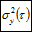#### File Exchange > Data Analysis >    Allan VarianceAuthor:
OriginLab Technical Support
8/11/2015
Last Update:
2/3/2023
129
Total Ratings:
6
File Size:
49 KB
Average Rating:File Name:
AllanVar.opx
File Version:
1.21
Minimum Versions:
Free
Type:
App
Summary:

Compute Allan variance for time series at given tau values.

Description:

PURPOSE
This app can be used to calculate Allan variance for a time series signal at given tau values. The output includes:

1. Allan deviation
2. Allan variance
3. Log-Log plot for Allan deviation
4. Angle random walk plot
5. Overlapping Allan deviation, modified Allan deviation, time Allan deviation, Hadamard  Allan deviation, overlapping Hadamard  Allan deviation.

INSTALLATION
Download AllanVar.opx file, and then drag-and-drop onto the Origin workspace. An icon will appear in the Apps Gallery window.
NOTE: This tool requires OriginPro.

OPERATION

1. Create a worksheet and input data. Next activate the worksheet and click the Allan Variance icon in the Apps Gallery window.
2. In the opened dialog's Input tab, select Data Type to specify whether input data is Phase or Frequency. Select the XY data from the worksheet as Input Data. A value shown in Sampling Interval is automatically calculated according to your X data.  Uncheck the Auto check box to the right of Sampling Interval and specify a value if you don't want to use the calculated value.
3. tau Values Type provides three options: All, Octave and Custom. All option will calculate the result for tau values from 1 to one-half of the number of input points. Octave option defines tau values by an exponent of base 2, i.e. $$\tau_0, 2\tau_0, 4\tau_0, 8\tau_0,$$... and so on.  Custom option will allow you to choose tau Values from the worksheet.
4. In the Graph tab, you can determine whether to show the Log-Log Plot for Allan deviation and add Angle Random Walk Plot.
5. In the Output tab, specify the destination for output results. Results include Allan Deviation, Allan Variance, Overlapping Allan Deviation, Modified Allan Deviation, Time Allan Deviation, Hadamard Allan Deviation and Overlapping Hadamard Allan Deviation.

ALGORITHM
For frequency input data y with N points, its time interval is dt, the Allan variance at $$\tau = m \ dt$$ can be calculated as follows:

• Divide y into P subgroups, $$P = N / m$$
• Calculate the mean value in each subgroup $$Y(1+(i-1)m), \; i=1,2,...P$$.
• Calculate the difference for mean values in adjacent subgroups.
• Allan variance is half of the mean square of these difference values.
• Allan deviation is the square root of Allan variance.

If input data is phase data x, it can be converted to frequency y first.

$$y = \frac {dx} {dt}$$

Other results can be calculated in a similar way.

• Overlapping Allan deviation
$$\sigma_y^2 (\tau) = \frac{1}{2(N-2m+1)} \displaystyle \sum_{i=1}^{N-m+1} \Big[ Y(i+m) - Y(i) \Big]^2$$
• Modified Allan deviation
$$\sigma_y^2 ( \tau ) = \frac{1}{ 2(N+2-3m)m^2 } \displaystyle \sum_{i=1}^{N-3m+2} \left \{\sum _{j=1}^{m} \Big[ Y(i+j+m-1) - Y(i+j-1) \Big] \right\}^2$$
• Time Allan deviation
$$\sigma_y^2 ( \tau ) = \left( \frac {\tau^2}{3} \right) \text{mdev}^2$$
$$\sigma_y^2 ( \tau ) = \frac{1}{6 (P-2) m} \displaystyle \sum_{i=1}^{P-2} \left (\sum_{j=1}^{m} \Big[ y( (i+1)m+j ) - 2y( i \cdot m + j) + y( (i-1)m + j ) \Big] \right )^2$$
$$\sigma_y^2 ( \tau ) = \frac{1}{6 (N-3m+1) m} \displaystyle \sum_{i=1}^{N-3m+1} \left (\sum_{j=1}^{m} \Big[ y( 2m+(i-1)+j ) - 2y( m + (i-1)+j) + y( i-1 + j ) \Big] \right )^2$$

REFERENCE

1. P. Werle, R. Mucke, F. Slemr. The Limits of Signal Averaging in Atmospheric Trace-Gas Monitoring by Tunable Diode-Laser Absorption Spectroscopy (TDLAS). Applied Physics B 57, 131-139 (1993).
2. Riley, W. and Howe, D. (2008), Handbook of Frequency Stability Analysis, Special Publication (NIST SP) - 1065, National Institute of Standards and Technology, Gaithersburg, MD

NOTE

1. This tool requires x data to be evenly spaced. If it is not, it will show a warning message at the bottom of the dialog. Precision error may cause ununiform x, and you can ignore the warning message caused by this error.
2. The Allan variance is calculated by the difference of values averaged over the measurement interval tau therefore phase data cannot be used in this tool directly.

 10/14/2022 OriginLab Hi idutta, We will consider adding error analysis in the future (/APPS-1219). Thanks, OriginLab 10/14/2022 idutta Its a very useful tool. Thanks for creating it. Is it possible to add the error analysis as well? Thanks10/11/2022 puspendub Really useful04/06/2022 HLL12333 Really useful！02/14/2018 jdf726 Ah... I got confused between the 'year' and the 'number' based Origin release system. My version is 9.3.226 64 bit. I looked at this versus the old version (which did install). From what I can gather looking at the content of this opx file (without being able to install it), the code that performs the actual calculation has been put into a separate compressed C file (unlike the older one, where it is all in one file I think). I didn't seem to be able to read this ocz file to see what the improvements were, or perhaps to extract a version that is compatible with my Origin install. I doubt that this function uses any features that Origin 2017 has but 2016 does not, so why the insistence on the latest Origin version?... 02/13/2018 jdf726 I have Origin 2016 Pro, but this won't install - says I need Origin 9.4 or greater.